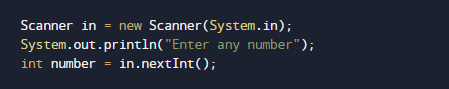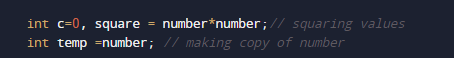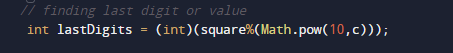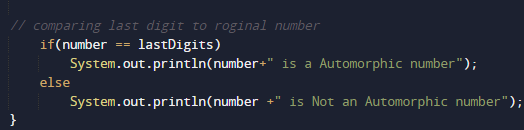# Program to check Automorphic Number in Java

In this tutorial you will learn about the Program to check Automorphic Number in Java and its application with practical example.

In this tutorial, we will learn to create a Java Program to check Automorphic Numbers in Java using Java programming.

## Prerequisites

Before starting with this tutorial we assume that you are best aware of the following Java programming topics:

• Java Operators.
• Basic Input and Output function in Java.
• Class and Object in Java.
• Basic Java programming.
• If-else statements in Java.
• For loop in Java.

## What is an automorphic number?

A number is called an automorphic number if square of the number ends with the same number itself as shown in example below.

Example. 5,6, 25, 76 etc  are automorphic numbers.## Program to check Automorphic Number in Java

In this our program we will find given number is a automorphic number or not in using java. We would first declared and initialized the required variables. Next, we would prompt user to input the a number.Later we  find number is automorphic  number or not let’s have a look at the code.

## Output

Automorphic numberNot a Automorphic Number.In the above program, we have first declared and initialized a set variables required in the program.

• number= it will hold entered number.
• temp= ti will actual copy of a number.
• lastDigits=it will hold last digit of square of given number.
• square=it will hols square of given number.

After declaring variables in the next statement user will be prompted to enter a value and  which will be assigned to variable ‘number’.And after that we will find square of that value and after that we will find last two digits of given number and making the copy of original number as shown in picture below.After that we will find last two digits of a number.then check condition for Automorphic  number if condition get satisfied it means the given number is Automorphic  number if not then it is not  Automorphic number.And finally we print the given number is Automorphic Number or not.

In this tutorial we have learn about the Program to check Automorphic Number in Java and its application with practical example. I hope you will like this tutorial.